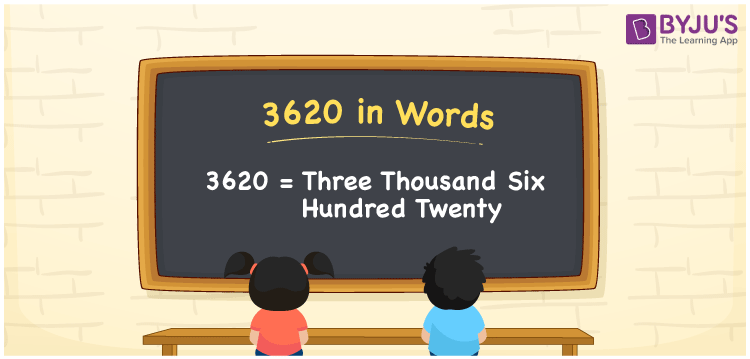# 3620 in words

3620 in words is written as Three Thousand Six Hundred and Twenty. 3620 represents the count or value. The article on Counting Numbers can give you an idea about count or counting. The number 3620 is used in expressions that relate to money, distance, length, year and others. Let us consider an example for 3620. “I purchased a table lamp that cost me Three Thousand Six Hundred and Twenty rupees.” Another example, “The first edition of a new weekly magazine sold Three Thousand Six Hundred and Twenty copies”

 3620 in words Three Thousand Six Hundred and Twenty Three Thousand Six Hundred and Twenty in Numbers 3620

## 3620 in English Words## How to Write 3620 in Words?

We can convert 3620 to words using a place value chart. The number 3620 has 4 digits, so let’s make a chart that shows the place value up to 4 digits.

 Thousands Hundreds Tens Ones 3 6 2 0

Thus, we can write the expanded form as:

3 × Thousand + 6 × Hundred + 2 × Ten + 0 × One

= 3 × 1000 + 6 × 100 + 2 × 10 + 0 × 1

= 3620

= Three Thousand Six Hundred and Twenty.

3620 is the natural number that is succeeded by 3619 and preceded by 3621.

3620 in words – Three Thousand Six Hundred and Twenty.

Is 3620 an odd number? – No.

Is 3620 an even number? – Yes.

Is 3620 a perfect square number? – No.

Is 3620 a perfect cube number? – No.

Is 3620 a prime number? – No.

Is 3620 a composite number? – Yes.

## Solved Example

1. Write the number 3620 in expanded form

Solution: 3 × 1000 + 6 × 100 + 2 × 10 + 0 × 1

We can write 3620 = 3000 + 600 + 20 + 0

= 3 × 1000 + 6 × 100 + 2 × 10 + 0 × 1

## Frequently Asked Questions on 3620 in words

Q1

### How to write 3620 in words?

3620 in words is written as Three Thousand Six Hundred and Twenty.
Q2

### State if True or False. 3620 is divisible by 2?

True. 3620 is divisible by 2.
Q3

### Is 3620 a perfect square number?

No. 3620 is not a perfect square number.